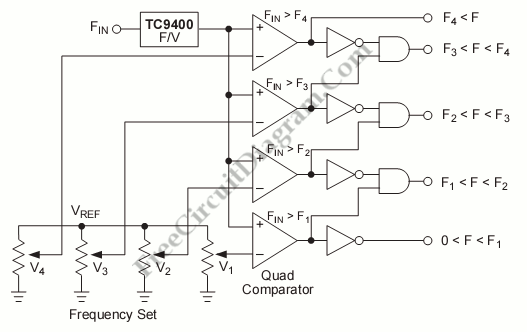## Logarithmic Amplifier Using MC1556 Op-Amp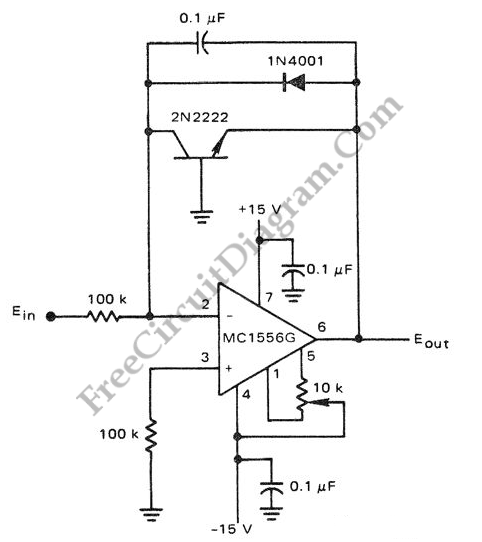The circuit below is a logarithmic amplifier using an MC1556 op amp. The 10K pot is an offset adjustment.  Here is  a schematic diagram of one classic example of  general purpose  logarithmic converter circuit employing LM1556 op-amp: The popularity of logarithmic amplifier is decreasing as the microprocessor and microcontroller chips are  getting lower and lower in price. Long time ago, […]

## AGC (Compressor) Speech Processor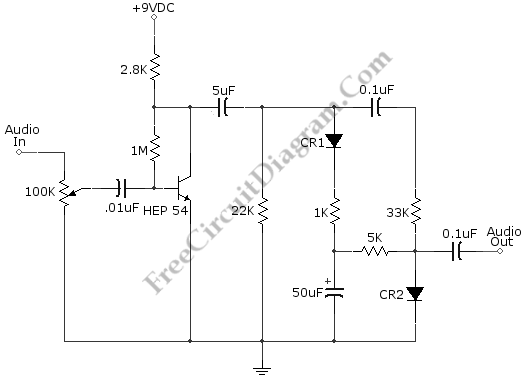This is a speech processor circuit that can be used in communication radio circuit. This circuit consist of two sections, they are amplification and compression or dipping. The combination of these processing will improve the  SSB communication intelligibility and provide higher average level while avoiding clipping. The 100K pot is used to adjust the compression degree, as well as adjusting […]

## Infinite Resolution Frequency Multiplier/Divider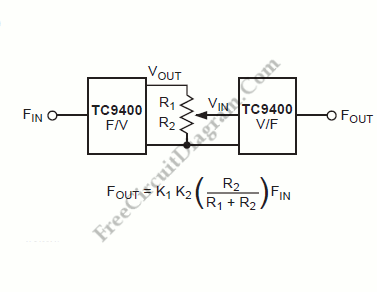Normally, frequency multiplier or divider has only discrete ratio operation since it uses digital divider as the main process for division or multiplication. By first converting the incoming frequency into a proportional DC voltage, we can perform frequency scaling easily with no restriction of discrete ratio operation. Here is the block diagram the scheme: We can accomplished it by using […]

## Accurate Low-Cost Square-Root Converter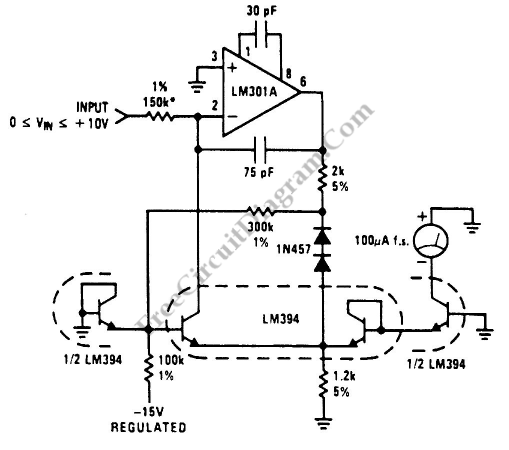This is a Low-Cost Accurate Square-Root Circuit. This circuit is used to provide a square-root function with a good accuracy. The benefit of This circuit is cheap. This circuit produce a current output which can be used to be converted to voltage by a summing junction current-to-voltage converter or to drive a meter directly. This circuit requires 15V supply. The […]

## Phase-Shift Meter for Audio Frequency Signal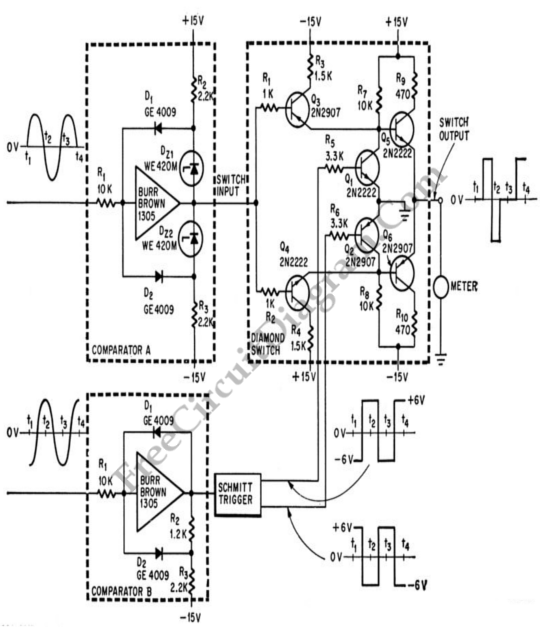Simple phase shift measurement can be done by squaring both the measured and the reference, then compute the difference of the two signals. The accuracy of the circuit shown in the schematic diagram  below is 1% UP to 2000 cps. This circuit is used in computers and for high speed analog instrumentation. Negative value for zero phase shift is indicated […]

## Mic/Guitar Compressor with Transitor Bias Control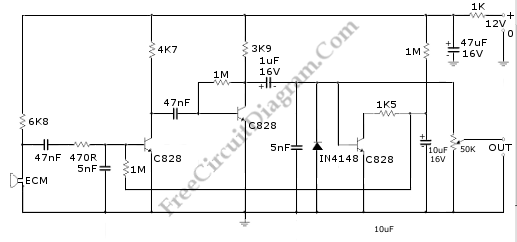This is a compressor circuit that can be used for dynamic mic, condenser mic, or electric guitar pickup. This circuit doesn’t produce very good sound output, but it has very stable amplitude/volume. This compressor circuit design is unique since it uses base current control for manipulating the gain. Here is the schematic diagram of the circuit: The first and second […]